Search by Topic

Resources tagged with Angles - points, lines and parallel lines similar to Quad in Quad:

Filter by: Content type:
Age range:
Challenge level:

There are 36 results

Broad Topics > Angles, Polygons, and Geometrical Proof > Angles - points, lines and parallel linesAge 14 to 16 Challenge Level:

Join the midpoints of a quadrilateral to get a new quadrilateral. What is special about it?Age 14 to 16 Challenge Level:

The points P, Q, R and S are the midpoints of the edges of a non-convex quadrilateral.What do you notice about the quadrilateral PQRS and its area?Similarly So

Age 14 to 16 Challenge Level:

ABCD is a square. P is the midpoint of AB and is joined to C. A line from D perpendicular to PC meets the line at the point Q. Prove AQ = AD.Parallel Universe

Age 14 to 16 Challenge Level:

An equilateral triangle is constructed on BC. A line QD is drawn, where Q is the midpoint of AC. Prove that AB // QD.Same Length

Age 11 to 16 Challenge Level:

Construct two equilateral triangles on a straight line. There are two lengths that look the same - can you prove it?Angle Trisection

Age 14 to 16 Challenge Level:

It is impossible to trisect an angle using only ruler and compasses but it can be done using a carpenter's square.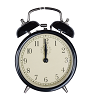On Time

Age 11 to 14 Challenge Level:

On a clock the three hands - the second, minute and hour hands - are on the same axis. How often in a 24 hour day will the second hand be parallel to either of the two other hands?Hand Swap

Age 14 to 16 Challenge Level:

My train left London between 6 a.m. and 7 a.m. and arrived in Paris between 9 a.m. and 10 a.m. At the start and end of the journey the hands on my watch were in exactly the same positions but the. . . .Polygon Rings

Age 11 to 14 Challenge Level:

Join pentagons together edge to edge. Will they form a ring?Tessellating Hexagons

Age 11 to 14 Challenge Level:

Which hexagons tessellate?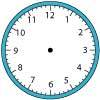Right Time

Age 11 to 14 Challenge Level:

At the time of writing the hour and minute hands of my clock are at right angles. How long will it be before they are at right angles again?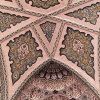Angles Inside

Age 11 to 14 Challenge Level:

Draw some angles inside a rectangle. What do you notice? Can you prove it?Shogi Shapes

Age 11 to 14 Challenge Level:

Shogi tiles can form interesting shapes and patterns... I wonder whether they fit together to make a ring?Right Angles

Age 11 to 14 Challenge Level:

Can you make a right-angled triangle on this peg-board by joining up three points round the edge?Semi-regular Tessellations

Age 11 to 16 Challenge Level:

Semi-regular tessellations combine two or more different regular polygons to fill the plane. Can you find all the semi-regular tessellations?Pythagoras

Age 7 to 14

Pythagoras of Samos was a Greek philosopher who lived from about 580 BC to about 500 BC. Find out about the important developments he made in mathematics, astronomy, and the theory of music.Triangles in Circles

Age 11 to 14 Challenge Level:

Can you find triangles on a 9-point circle? Can you work out their angles?Subtended Angles

Age 11 to 14 Challenge Level:

What is the relationship between the angle at the centre and the angles at the circumference, for angles which stand on the same arc? Can you prove it?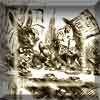A Problem of Time

Age 14 to 16 Challenge Level:

Consider a watch face which has identical hands and identical marks for the hours. It is opposite to a mirror. When is the time as read direct and in the mirror exactly the same between 6 and 7?Which Solids Can We Make?

Age 11 to 14 Challenge Level:

Interior angles can help us to work out which polygons will tessellate. Can we use similar ideas to predict which polygons combine to create semi-regular solids?Robotic Rotations

Age 11 to 16 Challenge Level:

How did the the rotation robot make these patterns?Making Sixty

Age 14 to 16 Challenge Level:

Why does this fold create an angle of sixty degrees?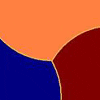Three Tears

Age 14 to 16 Challenge Level:

Construct this design using only compasses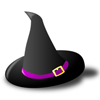Witch's Hat

Age 11 to 16 Challenge Level:

What shapes should Elly cut out to make a witch's hat? How can she make a taller hat?Making Maths: Equilateral Triangle Folding

Age 7 to 14 Challenge Level:

Make an equilateral triangle by folding paper and use it to make patterns of your own.Interacting with the Geometry of the Circle

Age 5 to 16

Jennifer Piggott and Charlie Gilderdale describe a free interactive circular geoboard environment that can lead learners to pose mathematical questions.Age 14 to 16 Challenge Level:

Make five different quadrilaterals on a nine-point pegboard, without using the centre peg. Work out the angles in each quadrilateral you make. Now, what other relationships you can see?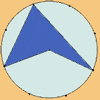Angle Measurement: an Opportunity for Equity

Age 11 to 16

Suggestions for worthwhile mathematical activity on the subject of angle measurement for all pupils.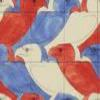Maurits Cornelius Escher

Age 7 to 14

Have you ever noticed how mathematical ideas are often used in patterns that we see all around us? This article describes the life of Escher who was a passionate believer that maths and art can be. . . .Orbiting Billiard Balls

Age 14 to 16 Challenge Level:

What angle is needed for a ball to do a circuit of the billiard table and then pass through its original position?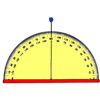Making Maths: Clinometer

Age 11 to 14 Challenge Level:

You can use a clinometer to measure the height of tall things that you can't possibly reach to the top of, Make a clinometer and use it to help you estimate the heights of tall objects.LOGO Challenge 7 - More Stars and Squares

Age 11 to 16 Challenge Level:

Can you use LOGO to create a systematic reproduction of a basic design? An introduction to variables in a familiar setting.LOGO Challenge 8 - Rhombi

Age 7 to 16 Challenge Level:

Explore patterns based on a rhombus. How can you enlarge the pattern - or explode it?Estimating Angles

Age 7 to 14 Challenge Level:

How good are you at estimating angles?Round and Round and Round

Age 11 to 14 Challenge Level:

Where will the point stop after it has turned through 30 000 degrees? I took out my calculator and typed 30 000 ÷ 360. How did this help?LOGO Challenge 1 - Star Square

Age 7 to 16 Challenge Level:

Can you use LOGO to create this star pattern made from squares. Only basic LOGO knowledge needed.